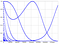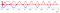# The Irrationality Measure of 𝜋 as Seen through the Eyes of Cosine

A while ago during my freshman year of undergraduate studies, I wrote a paper with my professor, Dr. Erin P.J. Pearse, detailing lengthy proofs on the relation between certain sequences of the trigonometric function cosine, and its relation to something known as the “irrationality measure” of 𝜋. A lot of my friends/peers get curious about this particular paper, because the bulk of it looks like unintelligible nonsense to the untrained eye. In reality, a lot of the concepts and results behind the paper are very approachable with as little as a high school level mathematics! The main theorem of the paper is as follows:

Granted, this is an intimidating chunk of math, but I really was serious that you only need a high school level education of mathematics to understand it! So let’s break this down, with a little background information to start.

# What kinds of numbers are there?

This sounds like a very broad question, and indeed it is, but there’s a relatively simple way to classify most numbers you’d encounter on a daily basis.

First, let’s start with the natural numbers. Natural numbers are basically the numbers you use to count arrangements of objects; I can have one apple, two apples, three apples… and so forth. Whether or not you include zero as a natural number is left mostly to personal preference — some regard the set of natural numbers along with zero as the whole numbers.

Next, we have the integers, which are basically the same as the naturals but include negative naturals as well (…-3, -2, -1, 0, 1, 2, 3…).

Another level up, we have the rational numbers: basically any number that can be represented as a fraction. Note that this includes all of the previously mentioned categories, since any integer or natural number can simply written as the number divided by 1 (e.g. 3 = 3/1, 123=123/1). It also includes many decimal numbers, specifically decimal numbers that terminate, or repeat periodically (e.g. 0.3333333… = 1/3, 0.0625 = 1/16).

On top of this, we have the irrational numbers, which are any decimal numbers that do not terminate or repeat periodically. These are a little harder to think about, because there’s no good real world analogy (when was the last time you had √2 apples?), but bear with me. For the sake of the rest of this article, just view irrational numbers as numbers that cannot be put in the form of a fraction (as the rationals can).

There are PLENTY more categories of numbers (algebraic, transcendental, complex, hypercomplex, transfinite, hyperreal, surreal… to name a few), and each really deserves an article of its own, but what we’ve defined so far is good enough.

# Getting Closer…

Now let’s talk about another important idea. Remember when I said that irrational numbers include all the numbers that can’t be represented by rational numbers (i.e. fractions)? Well, it turns out, for any irrational number, you can “pick” a rational number that is as close as you want to that irrational number. Let me show you what that means with a few examples.

Let’s take an irrational number, the square root of 2, which is 1.414213562… and try to find some rational numbers which are close to it. For example, 3/2 = 1.5 is pretty close to 1.41421356237…, in fact it’s only 6% different! But we can do better — infinitely better, actually. What if instead of 3/2, we picked something like 141/100=1.41? That’s only 0.03% away. What about 1414/1000? That’s 0.02% away! You can probably see where I’m going with this — basically, take as many numbers from the decimal representation of the irrational number you want to approximate, truncate it, and divide by the corresponding power of 10, and boom, you picked a rational number arbitrarily close to that irrational number!

# Some Irrational Numbers are More Rational than Others

We’ve just learned that we can approximate any irrational number arbitrarily well with any rational number, but that doesn’t mean that it’s efficient to do so for every number. Remember the example with the square root of 2? Let’s say we wanted to get within 0.0000002% of the square root of 2 using the method previously described. Well, we’d need to make a pretty nasty looking fraction to get there: 141421356/100000000. This isn’t the case for every number though! Take a look at this number: 1.100100001000000001…, which we construct by starting with 1.1, then adding two zeros and a one to the end (1.1001), then adding four zeroes and a one (1.100100001), then adding eight zeroes and a one (1.100100001000000001), and so forth to infinity. If we wanted to approximate this number to 0.0000002% accuracy, we could do it with a tiny fraction: 11001/10000.

So, it seems like some irrational numbers need “more complicated” fractions to approximate them well than others. In a sense, the less complicated fraction needed to approximate an irrational number, the more “rational” that number is, since that number just happens to be very close to some “nice” rational number. In fact, there is a precise mathematical definition of “irrationality measure,” which defines how rational a number is in the sense mentioned previously. The actual definition is a little complicated, and I encourage you to read more about it if you’re interested, but it essentially measures how the size of the denominator (lower part) of the fraction grows in size as the approximation of the irrational number gets better.

# Let’s Talk about Cosine Now

As you may remember from high school, cosine is a periodic function which can be defined, in short, by the x-coordinate of the unit circle at varying angles, as beautifully illustrated by the GIF below.

For those of you who remember a little more about cosine, you’ll recall that the value of cosine is only equal to 1 at angle values of 0, 2𝜋, 4𝜋, 6𝜋… and so on. Looking back at the unit circle diagram, this is because the only times cosine is flatly, positively horizontal, are at positions corresponding to zero degrees or integer multiples of 360° (2𝜋 radians).

For reasons that are a little beyond the scope of this article to prove (but are actually rather intuitive), there exists no integer value (other than zero) at which cosine is equal to 1 (e.g.: cos(1) ≈ 0.5403, cos(2) ≈ -0.4161 ,cos(3) ≈ -0.6536…). Yet, similar to the way in which we can approximate any irrational number arbitrarily well with rational numbers, we can pick an integer that brings cosine arbitrarily close to 1 (e.g.: cos(377) = 0.99996). This, as well, follows somewhat intuitively from what we have previously discussed. You may notice that a question very similar to what we’ve asked previously is arising: to get cosine really close to 1, how big of a number do we need to choose? For example, the cosine of 43042119 is so close to 1 that it only differs by about a billionth of a percent!

# A Somewhat Arbitrary Sequence

Now, let’s do something a little funny. Take the cosine of a number, n, and multiply the result by itself n times (i.e. cos(n)ⁿ). Remember that when you multiply a positive number less than 1 by another positive number less than one, the result is a number even smaller than the original numbers (e.g. 0.5*0.3 = 0.15). So certainly, if we took a number like cos(377), which is less than 1, and multiplied it by itself 377 times, we should end up with a really tiny number, right? Astonishingly, sometimes we don’t. In fact, cos(377)³⁷⁷ is approximately equal to 0.985, less than 2% different from cos(377).

This is a little surprising, and in fact we can find many other examples where this holds true! The numbers seem distributed somewhat randomly, but when we plot cos(n)ⁿ, something magical happens:This is one single plot, despite looking like a composition of many different curves!

What in the world is going on here? It looks kind of like a bunch of random curves all mixed together, yet it was produced from one single equation: cos(n)ⁿ. Furthermore, there seem to be no end to the number of integers we can pick in which cos(n)ⁿ is still pretty close to 1!Each red peak here is an integer that brings cos(n)ⁿ pretty close to 1. Notice that the scale of the x-axis is 100000 per tick!

And now, more questions arise: are there infinitely many numbers that bring cos(n)ⁿ pretty close to 1? If so, why? What are those weird curves in the first graph, and why do they appear? Can we isolate and describe those curves mathematically?

# It Gets Deeper…

In short, the answer to the previous questions, respectively, are: yes, it’s complicated, it’s complicated, and yes.

There are a multitude of theorems and proofs we describe in our paper that answer all of these questions in detail (check if out!), but they are rather involved, and again, out of the scope of this article. Rather, it is what we found in searching for these answers that is the true interest and focus of the paper. As mentioned earlier, we can choose infinitely many numbers that bring cos(n)ⁿ arbitrarily close to 1, but did you know you could also find infinitely many numbers that bring cos(n)^(n^1.5) close to 1? And even cos(n)^(n^1.99999) close to 1? Yet, we struggle to find many numbers that bring cos(n)^(n²) close to 1 (though we cannot prove this yet). Why??

What we’ve shown in this paper is that the irrationality measure of π is directly linked to whether or not we can choose infinitely many numbers that bring cos(n)^(n^something) very close to 1. In fact, we show that the irrationality measure of π is exactly equal to 2*something -2. Where something is the greatest number at which we can still find infinitely many numbers that bring cos(n)^(n^something) really close to 1.

There are other cool results that we prove, including the fact that we can choose infinitely many integers that bring cos(n)^(n²) arbitrarily close to about 0.6065, or that we can find arbitrarily long sequences of equally spaced integers (i.e. arithmetic progressions) that are really close to 1 under special conditions of our cosine sequence.

# Conclusion

I hope this sheds a little light on what the core of this paper is about, and how there can be incredible complexity underlying simple looking things, like cos(n)!

I could not have written this paper without the help of my incredibly brilliant professor, Dr. Erin P.J. Pearse, who is currently a professor of mathematics at California Polytechnic University, San Luis Obispo, and I’m immensely thankful that he took the time to mentor me and guide me through this process!

Machine learning, mathematics, medicine. I do research in biotech.